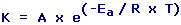Arrhenius Equation Calculator Enter value and click on calculate. Result will be displayed.K = Rate Constant A = Frequency Factor Ea = Activation Energy R = Universal Gas Constant = 8.314 x 10-3 kJ mol-1 K-1 T = Temperature
 Enter your values: Frequency Factor (A): Sec-1 Activation Energy (Ea): kJ Mol-1 Temperature (T): K Result: Rate Constant (K): Sec-1

 Web www.calculatoredge.com
 The equation was first proposed by the Dutch chemist J. H. van 't Hoff in 1884; five years later in 1889, the Swedish chemist Svante Arrhenius provided a physical justification and interpretation for it.

The Arrhenius equation is a simple, but remarkably accurate, formula for the temperature dependence of the rate constant, and therefore rate, of a chemical reaction.

At higher temperatures, the probability that two molecules will collide is higher. This higher collision rate results in a higher kinetic energy, which has an effect on the activation energy of the reaction. The activation energy is the amount of energy required to ensure that a reaction happens.

Gas constant comes from an equation, pV=nRT, which relates the pressure, volume and temperature of a particular number of moles of gas. Activation energy is the minimum energy needed for the reaction to occur.

Frequency Factor is a term which includes factors like the frequency of collisions and their orientation. It varies slightly with temperature, although not much. It is often taken as constant across small temperature ranges.

You can use the Arrhenius equation to show the effect of a change of temperature on the rate constant - and therefore on the rate of the reaction.LINKSDISCLAIMERCONTACT US